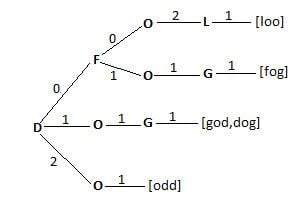Anatree

Updated onAn Anatree is a data structure designed to solve anagrams. Solving an anagram is the problem of finding a word from a given list of letters. These problems are commonly encountered in word games like wordwheel, scrabble or crossword puzzles that we find in newspapers. The problem for the wordwheel also has the condition that the central letter appear in all the words framed with the given set. Some other conditions may be introduced regarding the frequency (number of appearances) of each of the letters in the given input string. These problems are classified as Constraint satisfaction problem in computer science literature.

Contents

An anatree is represented as a directed tree which contains a set of words (W) encoded as strings in some alphabet. The internal vertices are labelled with some letter in the alphabet and the leaves contain words. The edges are labelled with non-negative integers. An anatree has the property that the sum of the edge labels from the root to the leaf is the length of the word stored at the leaf. If the internal vertices are labelled as α 1 , α 2 ... α l , and the edge labels are n 1 , n 2 ... n l , then the path from the root to the leaf along these vertices and edges are a list of words that contain n 1 α 1 s, n 2 α 2 s and so on. Anatrees are intended to be read only data structures with all the words available at construction time.

A Mixed Anatree is an anatree where the internal vertices also store words. A mixed anatree can have words of varying lengths, where as in a regular anatree, all words are of the same length.

Data Structures for solving Anagrams

A number of data structures have been proposed to solve anagrams in constant time. Two of the most commonly used data structures are the Alphabetic map and the Frequency map. The Alphabetic map maintains a hash table of all the possible words that can be in the language (this is referred to as the lexicon). For a given input sting, sort the letters in alphabetic order. This sorted string maps onto a word in the hash table. Hence finding the anagram requires sorting the letters and a looking up the word in the hash table. The sorting can be done in linear time by the counting sort and hash table look up can be done in constant time.

For example, for the word ANATREE, the alphabetic map would produce a mapping of { A A E E N R T > { a n a t r e e } } .

A Frequency map also stores the list of all possible words in the lexicon in a hash table. For a given input string, the frequency map maintains the frequencies (number of appearances) of all the letters and uses this count to perform a look up in the hash table. The worst case execution time is found to be linear in size of the lexicon.

For example, for the word ANATREE, the alphabetic map would produce a mapping of f ( A ) > 2 , f ( E ) > 2 , f ( N ) > 1 , f ( R ) > 1 , f ( T ) > 2 . The words that do not appear in the string are not written in the map.

Construction of an Anatree

The construction of an Anatree begins by selecting a label for the root and partitioning words based on the label chosen for the root. This process is repeated recursively for all the labels of the tree. Anatree construction is non-canonical for a given set of words, depending on the label chosen for the root, the anatree will differ accordingly. The performance of the anatree is greatly impacted by the choice of labels.

The following are some heuristics for choosing labels:

• Start labeling vertices in alphabetical order from the root. This approach reduces construction overhead
• Start labeling vertices based on the relative frequency. A probabilistic approach is used to assign labels to vertices. If W n α is the set of words that contain n α s , then we label the vertex with α if it maximizes the expected distance to the leaf. This approach has the most frequently appearing characters (like E) labeled at the root and the least frequently appearing characters labeled at the leaves. The following equation is maximized D α = n n | W n α | | W | . This approach prevents long sequences of zero labeled edges since they do not contribute letters to the words generated by the anatree.
• Finding Anagrams in an Anatree

To find a word in an anatree, start at the root, depending on the frequency of the label in the given input string, follow the edge that has that frequency till the leaf. The leaf contains the required word. For example, consider the anatree in the figure, to find the word d o g , the given string may be o g d . Start at the root and follow the edge that has 1 as the label. We follow this label since the given input string has 1 d . Traverse this edge until the leaf is encountered. That gives the required word.

Space and Time Requirements

A lexicon that stores w words (each word can be l characters long) in an alphabet A has the following space requirements.

The worst case execution time of an anatree is O ( | w | ( l + w | A | 2 ) )

References

Similar Topics
The Unscarred
Richard Bohringer
Andrew Ostrowski
Topics